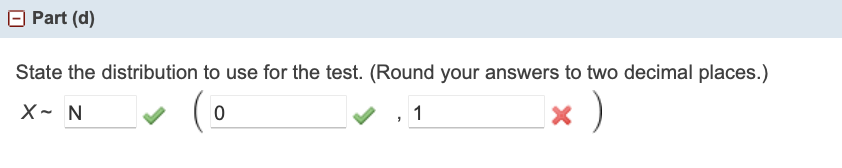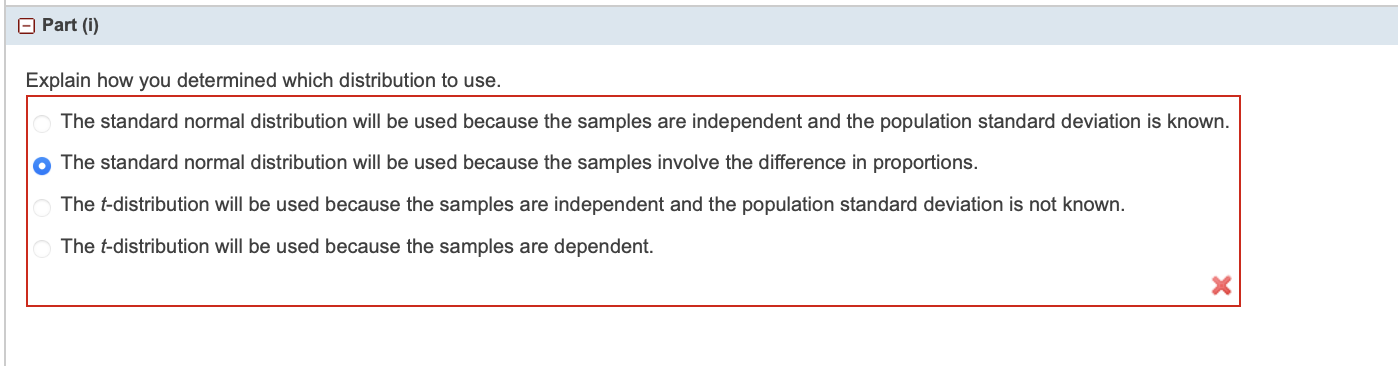Home / Expert Answers / Statistics and Probability / a-2-mmunity-college-and-u-four-year-university-state-the-distribution-to-use-for-the-test-ro-pa918

# (Solved): A-2 mmunity college and u= four-year university. State the distribution to use for the test. (Ro ...

A-2mmunity college and four-year university. State the distribution to use for the test. (Round your answers to two decimal places.) Explain how you determined which distribution to use. The standard normal distribution will be used because the samples are independent and the population standard deviation is known. The standard normal distribution will be used because the samples involve the difference in proportions. The -distribution will be used because the samples are independent and the population standard deviation is not known. The -distribution will be used because the samples are dependent.

We have an Answer from Expert